# Repetition & Selection 5

The following are the topics available in this part.

4. # Quick Reference

Using for Loops

The for loop is an alternative to the while loop. The flowchart in the following illustration shows a simple for loop.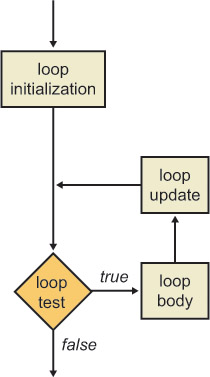The following example shows how to write a simple for loop in Visual C++. This example has exactly the same effect as the while loop you saw earlier.

for (int count = 1; count <= 5; count++)

{

Console::WriteLine(count * count);

}

Console::WriteLine(L"The end");

The parentheses after the for keyword contain three expressions separated by semicolons. The first expression performs loop initialization, such as setting loop counters. The initialization expression is performed only once, at the start of the loop. You can declare loop variables in the first expression of the for statement. The preceding example illustrates this technique. The count variable is local to the for statement and goes out of scope when the loop terminates. The second expression in the for statement defines a test. If the test evaluates to true, the loop body is executed. After the loop body has been executed, the final expression in the for statement is executed; this expression performs loop update operations, such as incrementing loop counters. The for statement is very flexible. You can omit any of the three expressions in the for construct as long as you retain the semicolon separators. You can even omit all three expressions, as in for( ; ; ), which represents an infinite loop. The preceding example displays the output shown in the following graphic.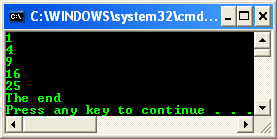In this exercise, you will modify your Calendar Assistant application so that it uses a for loop rather than a while loop to obtain five dates from the user.

Continue working with the project from the previous exercise.

Modify the code in the main() function to use a for loop rather than a while loop, as shown here:

int main(array<System::String ^> ^args)

{

for (int count = 1; count <= 5; count++)

{

Console::WriteLine(count);

int year = GetYear();

int month = GetMonth();

int day = GetDay(year, month);

DisplayDate(year, month, day);

}

return 0;

}

Notice that there is no count++ statement after display the date because the for statement takes care of incrementing the loop counter.

Build and run the program. The program asks you to enter five dates, as before.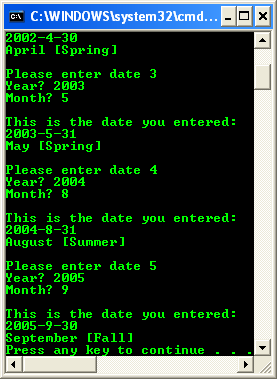Using do-while Loops

The third and final loop construct in Visual C++ is the do-while loop. The do-while loop is fundamentally different from the while loop and the for loop because the test comes at the end of the loop body, which means that the loop body is always executed at least once in a do-while loop. The following illustration shows a simple do-while loop.

-------------------------The following example shows how to write a simple do-while loop in Visual C++. This example generates random numbers between 1 and 6 inclusive to simulate a die, and counts how many throws are needed to get a 6.

Random ^ r = gcnew Random();

int randomNumber;

int throws = 0;

do

{

randomNumber = r->Next(1, 7);

Console::WriteLine(randomNumber);

throws++;

}

while (randomNumber != 6);

Console::Write(L"You took ");

Console::Write(throws);

Console::WriteLine(L" tries to get a 6");

The loop starts with the do keyword, followed by the loop body, followed by the while keyword and the test condition. A semicolon is required after the closing parenthesis of the test condition. The preceding example displays the output shown in the following graphic.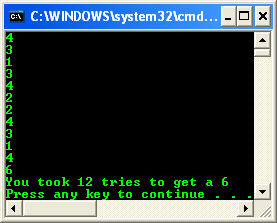In this exercise, you will modify your Calendar Assistant application so that it performs input validation, which is a typical use of the do-while loop.

Continue working with the project from the previous exercise.

Modify the GetMonth function as follows so that it forces the user to enter a valid month:

int GetMonth()

{

int month = 0;

do

{

Console::Write(L"Month [1 to 12]? ");

month = Convert::ToInt32(input);

}

while (month < 1 || month > 12);

return month;

}

Modify the GetDay() function as follows so that it forces the user to enter a valid day:

int GetDay(int year, int month)

{

int day = 0;

int maxDay;

if (month == 4 || month == 6 || month == 9 || month == 11)

{

maxDay = 30;

}

else if (month == 2)

{

bool isLeapYear = (year % 4 == 0 && year % 100 != 0) || (year % 400 == 0);

if (isLeapYear)

{

maxDay = 29;

}

else

{

maxDay = 28;

}

}

else

{

maxDay = 31;

}

do

{

Console::Write(L"Day [1 to ");

Console::Write(maxDay);

Console::Write(L"]? ");

day = Convert::ToInt32(input);

}

while (day < 1 || day > maxDay);

return day;

}

Build and run the program.

Try to enter an invalid month. The program keeps asking you to enter another month until you enter a value between 1 and 12, inclusive.

Try to enter an invalid day. The program keeps asking you to enter another day until you enter a valid number (which depends on your chosen year and month).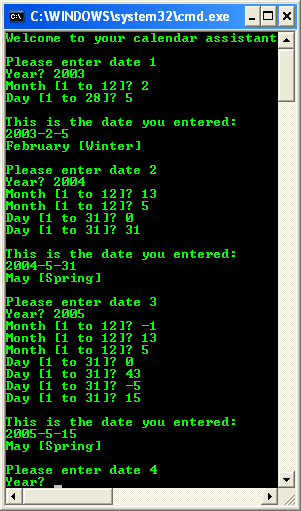Performing Unconditional Jumps

Visual C++ provides two keywords, break and continue, that enable you to jump unconditionally within a loop. The break statement causes you to exit the loop immediately. The continue statement abandons the current iteration and goes back to the top of the loop ready for the next iteration. The break and continue statements can make it difficult to understand the logical flow through a loop. Use break and continue sparingly to avoid complicating your code unnecessarily.

In this exercise, you will modify the main loop in your Calendar Assistant application. You will give the user the chance to break from the loop prematurely, skip the current date and continue on to the next one, or display the current date as normal.

Continue working with the project from the previous exercise.

Modify the main() function as follows to enable the user to break or continue if desired:

int main(array<System::String ^> ^args)

{

for (int count = 1; count <= 5; count++)

{

Console::WriteLine(count);

int year = GetYear();

int month = GetMonth();

int day = GetDay(year, month);

Console::Write(L"Press B (break), C (continue), or ");

Console::Write(L"anything else to display date ");

if (input->Equals(L"B"))

{

break;

}

else if (input->Equals(L"C"))

{

continue;

}

DisplayDate(year, month, day);

}

return 0;

}

Build and run the program.

After you have entered the first date, you will be asked whether you want to break or continue. Press X (or any other key except B or C) to display the date as normal.

Enter the second date, and then press C, which causes the continue statement to be executed. The continue statement abandons the current iteration without displaying your date. Instead, you are asked to enter the third date.

Enter the third date, and then press B, which causes the break statement to be executed. The break statement terminates the entire loop.Quick Reference

 To Do this Perform a one-way test. Use the if keyword followed by a test enclosed in parentheses. You must enclose the if body in braces if it contains more than one statement. For example:   if (n < 0)     {         Console::Write(L"The number ");         Console::Write(n);         Console::WriteLine(L" is negative");     } Perform a two-way test. Use an if-else construct. For example:   if (n < 0)     {         Console::Write(L"Negative");     }     else     {         Console::Write(L"Not negative");     } Perform a multiway test. Use an if-else-if construct. For example:   if (n < 0)     {         Console::Write(L"Negative");     }     else if (n == 0)     {         Console::Write(L"Zero");     }     else     {         Console::Write(L"Positive");     } Test a single expression against a finite set of constant values. Use the switch keyword followed by an integral expression enclosed in parentheses. Define case branches for each value you want to test against, and define a default branch for all other values. Use the break statement to close a branch. For example:   int dayNumber; // 0=Sun, 1=Mon, etc. // ... switch (dayNumber) { case 0: case 6:   Console::Write(L"Weekend");   break; default:   Console::Write(L"Weekday");   break; } Perform iteration by using the while loop. Use the while keyword followed by a test enclosed in parentheses. For example:   int n = 10; while (n >= 0) {    Console::WriteLine(n);    n--; } Perform iteration by using the for loop. Use the for keyword followed by a pair of parentheses. Within the parentheses, define an initialization expression, followed by a test expression, followed by an update expression. Use semicolons to separate these expressions. For example:   for (int n = 10; n >= 0; n--) {    Console::WriteLine(n); } Perform iteration by using the do-while loop. Use the do keyword, followed by the loop body, followed by the while keyword and the test condition. Terminate the loop with a semicolon. For example:   int n; do {   String ^ input = Console::ReadLine();   n = Convert::ToInt32(input); } while (n > 100); Terminate a loop prematurely. Use the break statement inside any loop. For example:   for (int n = 0; n < 1000; n++) {    int square = n * n;    if (square > 3500)    {      break;    }    Console::WriteLine(square); } Abandon a loop iteration and continue with the next iteration. Use the continue statement inside any loop. For example:   for (int n = 0; n < 1000; n++) {    int square = n * n;    if (square % 2 == 0)    {       continue;    }       Console::WriteLine(square); } Table 2